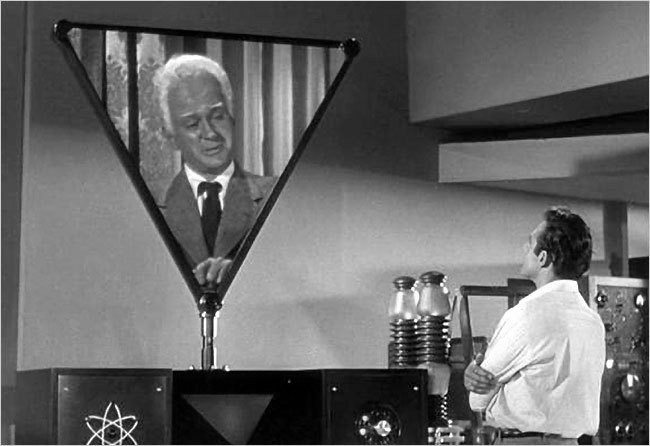Philosophy

# Bayesian Statistics Isn’t What You ThinkA Logical Probabilist (note the large forehead) explains that the interocitor has three states.

Back to our Edge series. Sean Carroll says Bayes’s Theorem should be better known. He outlines the theorem in the familiar updating-prior-belief formula. But, as this modified classic article shows, this is not the most important facet of Bayesian theory.

Below we learn all probabilities fit into the schema$\Pr(\mbox{Y}|\mbox{X})$, where X is the totality of evidence we have for proposition Y. It does not matter how this final number is computed (if indeed it can be): it can sometimes be computed directly, and sometimes by busting X apart into “prior” and “new” information, or sometimes by busting X apart into ways that are convenient for the mechanics of the calculation. That’s all Bayes’s theorem is: a way to ease calculation in some but not all instances. An example is given below. The real innovation—the real magic—comes in understanding all probability is conditional, i.e. that it fits into the schema. As shown in this talk-of-the-town book.

This post is modified version of one that was been restored after The Hacking. All original comments were lost.

Bayesian theory probably isn’t what you think. Most have the idea that it’s all about “prior beliefs” and “updating” probabilities, or perhaps a way of encapsulating “feelings” quantitatively. The real innovation is something much more profound. And really, when it comes down to it, Bayes’s theorem isn’t even necessary for Bayesian theory. Here’s why.

Any probability is denoted by the schematic equation$\Pr(\mbox{Y}|\mbox{X})$ (all probability is conditional), which is the probability the proposition Y is true given the premise X. X may be compound, complex or simple. Bayes’s theorem looks like this:$\Pr(\mbox{Y}|\mbox{W}\mbox{X}) = \frac{\Pr(\mbox{W}|\mbox{YX})\Pr(\mbox{Y}|\mbox{X})}{\Pr(\mbox{W}|\mbox{X})}$.
We start knowing or accepting the premise X, then later assume or learn W, and are able to calculate, or “update”, the probability of Y given this new information WX (read as “W and X are true or assumed true”). Bayes’s theorem is a way to compute$\Pr(\mbox{Y}|\mbox{W}\mbox{X})$. But it isn’t strictly needed. We could compute$\Pr(\mbox{Y}|\mbox{W}\mbox{X})$ directly from knowledge of W and X themselves. Sometimes the use of Bayes’s theorem can hinder.

Given X = “This machine must take one of states S1, S2, or S3”, we want the probability Y = “The machine is in state S1.” The deduced answer is 1/3. We then learn W = “The machine is malfunctioning and cannot take state S3”. The probability of Y given W and X is deduced as 1/2, as is trivial to see.

Now let’s find the result by applying Bayes’s theorem, the results of which must match. We know that$\Pr(\mbox{W}|\mbox{YX})/\Pr(\mbox{W}|\mbox{X}) = 3/2$, because$\Pr(\mbox{Y}|\mbox{X}) = 1/3$. But it’s difficult at first to tell how this comes about. What exactly is \Pr(\mbox{W}|\mbox{X}), the probability the machine malfunctions such that it cannot take state S3 given only the knowledge that it must take one of S1, S2, or S3? If we argue that if the machine is going to malfunction, given the premises we have (X), it is equally likely to be any of the three states, thus the probability is 1/3. Then$\Pr(\mbox{W}|\mbox{YX})$ must equal 1/2, but why? Given we know the machine is in state S1, and that it can take any of the three, the probability state S3 is the malfunction is 1/2, because we know the malfunctioning state cannot be S1, but can be S2 or S3. Using Bayes works, as it must, but in this case it added considerably to the burden of the calculation. In Uncertainty, I have other examples.

Most scientific, which is to say empirical, propositions start with the premise that they are contingent. This knowledge is usually left tacit; it rarely (or never) appears in equations. But it could: we could compute$\Pr(\mbox{Y}|\mbox{Y is contingent})$, which even is quantifiable (the open interval (0,1)). We then “update” this to$\Pr(\mbox{Y}|\mbox{X \& Y is contingent})$, which is 1/3 as above. Bayes’s theorem is again not needed.

Of course, there are many instances in which Bayes facilitates. Without this tool we would be more than hard pressed to calculate some probabilities. But the point is the theorem can but doesn’t have to be invoked as a computational aide. The theorem is not the philosophy.

The real innovation in Bayesian philosophy, whether it is recognized or not, came with the idea that any uncertain proposition can and must be assigned a probability, not in how the probabilities are calculated. (This dictum is not always assiduously followed.) This is contrasted with frequentist theory which assigns probabilities to some unknown propositions while forbidding this assignment in others, and where the choice is ad hoc. Given premises, a Bayesian can and does put a probability on the truth of an hypothesis (which is a proposition), a frequentist cannot—at least not formally. Mistakes and misinterpretations made by users of frequentist theory are legion.

The problem with both philosophies is misdirection, the unreasonable fascination with questions nobody asks, which is to say, the peculiar preoccupation with parameters. About that, another time.

Categories: Philosophy, Statistics

### 13 replies »

1.DAV says:

You do realize that persistence with these outlooks will only delay the employment offer from the Department of Statistical Anomalies. yes? It may however increase your prospects if another Librarian opening should arise at the Library.

2.Ye Olde Statistician says:

A cryptic reference by DAV, known only to devotees of the Library, the chief Librarian of which has an auspicious name.

3.John B() says:

Dr. Horace Worblehat?

4.Hal44 says:

Why haven’t the big forehead people conquered us by now?